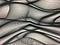# Grubb’s Test For OutliersPhoto by Armand Khoury on Unsplash

What is Grubb’s Test?

Grubb’s test is a statistical method to identify an outlier in a univariate dataset that follows a roughly Normal distribution.

In simple terms, a Normal distribution is if you drew a smooth line across your histogram, and it makes one symmetric mound. You most likely are dealing with a Normal Distribution (this is a generalized way of putting it).

This is an example of a Normal distribution:

Let’s assume we are consulting for a small business,and they have asked us to look over their monthly sales data and find A potential anomaly. Remember the Grubb’s test only finds ONE outlier.

Using a random number generator I came up with

[150, 175, 195, 161, 141, 199, 169, 174, 119, 100, 177, 156, 198, 186, 157, 134, 162, 125, 131, 128, 181, 112, 147, 122, 152, 115, 171, 186, 163, 104]

Since the data is approximatley normal we are ready to begin our calculations.

The minimum of this dataset is 100. Now let’s see if the day the store sold only a \$100 worth of items is an outlier.

The average of the dataset is 153.0

The standard deviation of the dataset is 28.83

After we determine the mean, standard deviation, and supposed outlier. We are ready to determine whether the data point is valid.

First, we have to get the G-statistic (which is similar to the Z-statistic or the T-statistic)

*We assume the minimum value to be our outlier (100)

Our G value is 1.838

Now, we just compare our G-value (1.838) to the value from a G-table — a predetermined chart with the threshold values for our G-statistics based on the Confidence Level.

If we slide our fingers carefully we can see that the G-table value for a “n” of 30 (dataset has 30 values) and a 95% confidence is 2.745.

For the last step, if the G-table value is less than the G-statistic we have calculated then the chosen outlier (the minimum for us) would be officially deemed as an outlier.

Since our G value of 1.838 is less than the G-table threshold of 2.745, \$100 is not an outlier in the monthly sales dataset.

Put simply,

G-stat > G-table value → the data point is an outlier

G-stat < G-table value → the data point is NOT an outlier

So we conclude the sales look good this month and there is nothing out of whack.

It’s that simple.

https://www.buymeacoffee.com/adarshbulusu

## More from Adarsh Bulusu

Innovation Enthusiast.